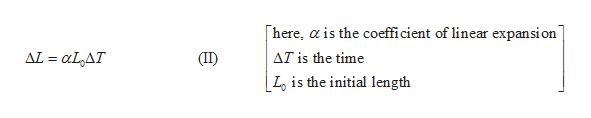# A thin rod consists of two parts joined together. Two-fifths of it is silver and three-fifths is gold. The temperature decreases by 27 C°.ΔL/(L0,Silver + L0,Gold)Determine the fractional decrease in the rod's length using the formula above, where L0,Silver and L0,Gold are the initial lengths of the silver and gold rods.

Question
27 views

A thin rod consists of two parts joined together. Two-fifths of it is silver and three-fifths is gold. The temperature decreases by 27 C°.

ΔL/(L0,Silver + L0,Gold)

Determine the fractional decrease in the rod's length using the formula above, where L0,Silver and L0,Gold are the initial lengths of the silver and gold rods.

check_circle

Step 1

The fractional decrease in length of the rod=∆L

The initial length of the silver rod=L0,silver

The initial length of the gold rod= L0,gold

The equation for fractional decrease in length is.

Step 2

The total decrease in length is equal to the sum of the decrease in length of both gold and silver rod.

Step 3

Write the expression for the decrease in length in terms of coefficient...help_outlineImage Transcriptionclosehere, a is the coefficient of linear expansion AL aLAT (II) AT is the time Lo is the initial length fullscreen

### Want to see the full answer?

See Solution

#### Want to see this answer and more?

Solutions are written by subject experts who are available 24/7. Questions are typically answered within 1 hour.*

See Solution
*Response times may vary by subject and question.
Tagged in

### Physics